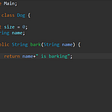# Introduction to Merge Sort Algorithm: How It Works and Why It’s So Efficient

The Merge Sort is one of the most efficient sorting algorithms. It is based on the divide-and-conquer method. In this article, I am going to explain how the algorithm works and why it is so efficient.

The merge sort algorithm belongs to the family of efficient sorting algorithms whose average complexity is O(n log(n)). This is the best performance that can be achieved with a comparison-based algorithm.

It is also an example of a divide-and-conquer method. This method solves a problem recursively, applying the three steps of recursion:

• Divide the problem into a number of small subproblems.
• Conquer the subproblems by solving them recursively.
• Combine the solution of each subproblem into the solution for the original problem.

# The algorithm, explained

First of all, let’s quickly review a few of the terms we use in recursion. If the subproblem of interest is large enough so that we need to make subsequent recursive calls, we call it a recursive case. When there are no more recursive calls to make, we call it the base case.

And here’s how merge sort works:

(Base case) If the sequence length is either 0 and 1, it’s ordered, indeed an array with just one element or zero element is ordered.

(Divide) Else we divide the sequence into two half subsequences. (Until the size of the subsequences are either zero or one → base case)

(Conquer) Each of these subsequences will be ordered, applying the same algorithm recursively.

(Combine) Once we have sorted two adjacent subsequences, we combine them together to get a sorted subsequence with a greater length, until we get the entire array sorted. In order to do that, we compare the first element of the two subsequences we are combining, the smaller one becomes the first element of the new output sequence (removing it from its subsequence). We repeat the procedure until both the subsequences are empty and the new output sequence covers all the elements of both the previous subsequences.

An example of this is provided in the how it works section.

The algorithm was invented by John von Neumann in the year 1945.

# How it works

First of all, we need to divide the input array iteratively into equal halves unless we have all subarray with length 1.

Once each array has only one element, it is obviously “sorted”.

We can now compare them in exactly the same manner that they were broken down: we compare the element for each list and then we combine them a new array in a sorted manner.

Let’s consider an array A and divide it into subarrays (A1, A2,…An) until we obtain the An array with only one element.

Then we split it into two subarrays: left subarray and right subarray.

We go on splitting since the length of the subarray is greater than 1…

Now it’s time to merge!

We are going to merge each pair of subarrays recursively to produce a newly sorted subarray.

In this way, subarrays are merged to produce a longer sorted subarray until we reach the sorted array.

And here’s how we merge two subarrays:

1. Compare the first element of the subarray A and the first element of subarray B.
2. Select the smallest element
3. Insert it into the resulting array

For example, let’s consider subarray A = { 2, 8, 11} and subarray B = { 5, 6, 28} with three element each one.

We have to obtain the ordered resulting array R = { 2, 5, 6, 8, 11, 28}.

Let’s apply the merge algorithm and see what’s happening:

# Pseudocode

Generalizing we can distingue two phases:

Split phase

• If the (sub)array has length 1 → stop the split phase
• If the (sub)array has length > than 1 → split in two parts (left and right)

`function mergeSort(array)`

`//If the length(array)=1 --> stop the split phase`

`if(lenght(array)==1)`

`return array`

`//Else split in two parts (left and right), go ahead splitting and then Merge`

`else`

`split(array) = [left, right];`

`left = mergeSort(left)`

`right = mergeSort(right)`

`array = Merge(left, right)`

`return array`

Merge phase

Merge the left and right (sub)array into a unique ordered array.

`function Merge(left, right)`

`//Merge the list until at least one of them is empty`

`while notEmpty(left) and notEmpty(right)`

`//If the element of the array left is less or equal to the first of right, put it in the result array`

`if first(left) <= first(right)`

`append first(left) to result`

`//Else put the first right array element in the result array`

`else`

`append first(right) to result`

# Programming languages implementation

C++

We are going to write two functions.

mergeSort

`void mergeSort(int array[], int first, int last){ //If the array contains only one element stop splitting if(first>=last) return; //Else go ahead splitting //Find the approximate middle //This formula is equal to (first+last)/2 //But avoids overflow for large first and last  int middle = first + (last-first)/2; //Go ahead splitting the left side mergeSort(array, first, middle); //Go ahead splitting the right side mergeSort(array, middle+1, last); //Then merge them merge(array, first, middle, last);}`

merge

`void merge(int array[], int first, int middle, int last){ //Calculate the lenth of the support array tmp int length = last-first+1;int tmp[length];int startLeft = first;int startRight = middle+1;for(int i = 0; i<length; ++i){if(startLeft>middle){tmp[i] = array[startRight++];}else if(startRight>last){tmp[i] = array[startLeft++];}else if(array[startLeft]<=array[startRight]){tmp[i] = array[startLeft++];}else{tmp[i] = array[startRight++];}}for (int m=0; m< length; ++m){array[first++] =tmp[m];}}`

The full code is available on my GitHub

# Properties

The Merge Sort is the best sorting algorithms for a large amount of data.

It is a stable algorithm, but it’s not adaptative.

We can use it in every situation, in particular when we want to order linked list and when random access is much more expensive than sequential access.

# Complexity

The merge sort is a really efficient algorithm for a large amount of input.

The time complexity can be expressed as following recurrence relation

`T(n) = 2T(n/2) + Θ(n)`

The complexity is Θ(n log(n)) in each case (average, worst, best).

--

--

--

## More from Sorting Algorithms

In Computer Science and Computer Engineering a very common problem is to sort a list in a certain order. Therefore, efficient sorting is crucial to optimise our software and we can accomplish that through smart sorting algorithms.

## Work From Home During Coronavirus Pandemic (Contribute Indonesia Government to Avoid COVID-19)## Spring Boot Quartz Scheduler with MySQL Database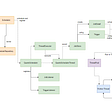## 4EVERLAND Provides One-Stop Dweb Solution for Harmony Ecosystem## Easily Automate a Table in SSMS## Gencove CLI v2.0.24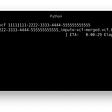## TryHackMe | Frank & Herby Make an App Writeup## Kubernetes Container Security## Andrea Cappelletti

Make it inspire 🌐

## Dynamic Programming On Grids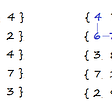## [LeetCode] (Easy) 118. Pascal’s Triangle 119. Pascal’s Triangle II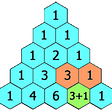## Behavior of the objects.